### IMO Shortlist 1982 problem 13

Kvaliteta:
Avg: 0.0
Težina:
Avg: 0.0
A non-isosceles triangle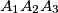$A_{1}A_{2}A_{3}$ has sides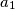$a_{1}$,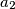$a_{2}$,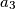$a_{3}$ with the side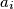$a_{i}$ lying opposite to the vertex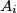$A_{i}$. Let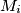$M_{i}$ be the midpoint of the side$a_{i}$, and let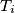$T_{i}$ be the point where the inscribed circle of triangle$A_{1}A_{2}A_{3}$ touches the side$a_{i}$. Denote by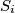$S_{i}$ the reflection of the point$T_{i}$ in the interior angle bisector of the angle$A_{i}$. Prove that the lines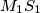$M_{1}S_{1}$,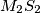$M_{2}S_{2}$ and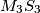$M_{3}S_{3}$ are concurrent.
Source: Međunarodna matematička olimpijada, shortlist 1982